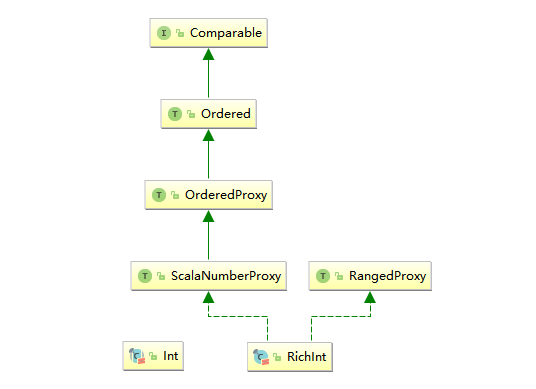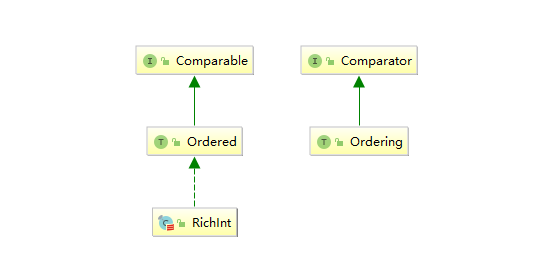# 大数据基础---Scala类型参数

## 一、泛型

Scala 支持类型参数化，使得我们能够编写泛型程序。

### 1.1 泛型类

Java 中使用 <> 符号来包含定义的类型参数，Scala 则使用 []

class Pair[T, S](val first: T, val second: S) {
override def toString: String = first + ":" + second
}

object ScalaApp extends App {

// 使用时候你直接指定参数类型，也可以不指定，由程序自动推断
val pair01 = new Pair("heibai01", 22)
val pair02 = new Pair[String,Int]("heibai02", 33)

println(pair01)
println(pair02)
}


### 1.2 泛型方法

object Utils {
def getHalf[T](a: Array[T]): Int = a.length / 2
}


## 二、类型限定

### 2.1 类型上界限定

Scala 和 Java 一样，对于对象之间进行大小比较，要求被比较的对象实现 java.lang.Comparable 接口。所以如果想对泛型进行比较，需要限定类型上界为 java.lang.Comparable，语法为  S <: T，代表类型 S 是类型 T 的子类或其本身。示例如下：

// 使用 <: 符号，限定 T 必须是 Comparable[T]的子类型
class Pair[T <: Comparable[T]](val first: T, val second: T) {
// 返回较小的值
def smaller: T = if (first.compareTo(second) < 0) first else second
}

// 测试代码
val pair = new Pair("abc", "abcd")
println(pair.smaller) // 输出 abc


public class Pair<T extends Comparable<T>> {
private T first;
private T second;
Pair(T first, T second) {
this.first = first;
this.second = second;
}
public T smaller() {
return first.compareTo(second) < 0 ? first : second;
}
}


### 2.2 视图界定

val pair1 = new Pair(10, 12)
val pair2 = new Pair(10.0, 12.0)


// 除了 compareTo 方法外，还提供了额外的关系符方法
trait Ordered[A] extends Any with java.lang.Comparable[A] {
def compare(that: A): Int
def <  (that: A): Boolean = (this compare that) <  0
def >  (that: A): Boolean = (this compare that) >  0
def <= (that: A): Boolean = (this compare that) <= 0
def >= (that: A): Boolean = (this compare that) >= 0
def compareTo(that: A): Int = compare(that)
}


// Predef.scala
@inline implicit def intWrapper(x: Int)   = new runtime.RichInt(x)// 视图界定符号 <%
class Pair[T <% Comparable[T]](val first: T, val second: T) {
// 返回较小的值
def smaller: T = if (first.compareTo(second) < 0) first else second
}


// 隐式转换为 Ordered[T]
class Pair[T <% Ordered[T]](val first: T, val second: T) {
def smaller: T = if (first.compareTo(second) < 0) first else second
}


### 2.3 类型约束

 // 1.使用隐式参数隐式转换为 Comparable[T]
class Pair[T](val first: T, val second: T)(implicit ev: T => Comparable[T])
def smaller: T = if (first.compareTo(second) < 0) first else second
}

// 2.由于直接继承 Java 中 Comparable 接口的是特质 Ordered，所以也可以隐式转换为 Ordered[T]
class Pair[T](val first: T, val second: T)(implicit ev: T => Ordered[T]) {
def smaller: T = if (first.compareTo(second) < 0) first else second
}


object PairUtils{
def smaller[T](a: T, b: T)(implicit order: T => Ordered[T]) = if (a < b) a else b
}


### 2.4 上下文界定

class Pair[T](val first: T, val second: T) {
// 请注意 这个地方用的是 Ordering[T]，而上面视图界定和类型约束，用的是 Ordered[T]，两者的区别会在后文给出解释
def smaller(implicit ord: Ordering[T]): T = if (ord.compare(first, second) < 0) first else second
}

// 测试
val pair= new Pair(88, 66)
println(pair.smaller)  //输出：66


// 1.定义一个人员类
class Person(val name: String, val age: Int) {
override def toString: String = name + ":" + age
}

// 2.继承 Ordering[T],实现自定义比较器,按照自己的规则重写比较方法
class PersonOrdering extends Ordering[Person] {
override def compare(x: Person, y: Person): Int = if (x.age > y.age) 1 else -1
}

class Pair[T](val first: T, val second: T) {
def smaller(implicit ord: Ordering[T]): T = if (ord.compare(first, second) < 0) first else second
}

object ScalaApp extends App {

val pair = new Pair(new Person("hei", 88), new Person("bai", 66))
// 3.定义隐式默认值,如果不定义,则下一行代码无法通过编译
implicit val ImpPersonOrdering = new PersonOrdering
println(pair.smaller) //输出： bai:66
}


### 2.5 ClassTag上下文界定

object ScalaApp extends App {
def makePair[T](first: T, second: T) = {
// 创建以一个数组 并赋值
val r = new Array[T](2); r(0) = first; r(1) = second; r
}
}


Scala 针对这个问题，提供了 ClassTag 上下文界定，即把泛型的信息存储在 ClassTag 中，这样在运行阶段需要时，只需要从 ClassTag 中进行获取即可。其语法为 T : ClassTag，示例如下：

import scala.reflect._
object ScalaApp extends App {
def makePair[T : ClassTag](first: T, second: T) = {
val r = new Array[T](2); r(0) = first; r(1) = second; r
}
}


### 2.6 类型下界限定

2.1 小节介绍了类型上界的限定，Scala 同时也支持下界的限定，语法为：U >: T，即 U 必须是类型 T 的超类或本身。

// 首席执行官
class CEO

// 部门经理
class Manager extends CEO

// 本公司普通员工
class Employee extends Manager

// 其他公司人员
class OtherCompany

object ScalaApp extends App {

// 限定：只有本公司部门经理以上人员才能获取权限
def Check[T >: Manager](t: T): T = {
println("获得审核权限")
t
}

// 错误写法: 省略泛型参数后,以下所有人都能获得权限,显然这是不正确的
Check(new CEO)
Check(new Manager)
Check(new Employee)
Check(new OtherCompany)

// 正确写法,传入泛型参数
Check[CEO](new CEO)
Check[Manager](new Manager)
/*
* 以下两条语句无法通过编译,异常信息为:
* do not conform to method Check's type parameter bounds(不符合方法 Check 的类型参数边界)
* 这种情况就完成了下界限制，即只有本公司经理及以上的人员才能获得审核权限
*/
Check[Employee](new Employee)
Check[OtherCompany](new OtherCompany)
}


### 2.7 多重界定

• 类型变量可以同时有上界和下界。 写法为 ：T > : Lower <: Upper

• 不能同时有多个上界或多个下界 。但可以要求一个类型实现多个特质，写法为 :

T < : Comparable[T] with Serializable with Cloneable

• 你可以有多个上下文界定，写法为 T : Ordering : ClassTag

## 三、Ordering & Ordered

• Comparable：可以理解为内置的比较器，实现此接口的对象可以与自身进行比较；
• Comparator：可以理解为外置的比较器；当对象自身并没有定义比较规则的时候，可以传入外部比较器进行比较。### 3.1 Comparable

import java.util.Arrays;
// 实现 Comparable 接口
public class Person implements Comparable<Person> {

private String name;
private int age;

Person(String name,int age) {this.name=name;this.age=age;}
@Override
public String toString() { return name+":"+age; }

// 核心的方法是重写比较规则，按照年龄进行排序
@Override
public int compareTo(Person person) {
return this.age - person.age;
}

public static void main(String[] args) {
Person[] peoples= {new Person("hei", 66), new Person("bai", 55), new Person("ying", 77)};
Arrays.sort(peoples);
Arrays.stream(peoples).forEach(System.out::println);
}
}

bai:55
hei:66
ying:77


### 3.2 Comparator

import java.util.Arrays;
import java.util.Comparator;

public class Person {

private String name;
private int age;

Person(String name,int age) {this.name=name;this.age=age;}
@Override
public String toString() { return name+":"+age; }

public static void main(String[] args) {
Person[] peoples= {new Person("hei", 66), new Person("bai", 55), new Person("ying", 77)};
// 这里为了直观直接使用匿名内部类,实现 Comparator 接口
//如果是 Java8 你也可以写成 Arrays.sort(peoples, Comparator.comparingInt(o -> o.age));
Arrays.sort(peoples, new Comparator<Person>() {
@Override
public int compare(Person o1, Person o2) {
return o1.age-o2.age;
}
});
Arrays.stream(peoples).forEach(System.out::println);
}
}


// 按照年龄大小排序
Arrays.sort(peoples, Comparator.comparingInt(o -> o.age));
Arrays.stream(peoples).forEach(System.out::println);
// 按照名字长度倒序排列
Arrays.sort(peoples, Comparator.comparingInt(o -> -o.name.length()));
Arrays.stream(peoples).forEach(System.out::println);


### 3.3 上下文界定的优点

// 1.定义一个人员类
class Person(val name: String, val age: Int) {
override def toString: String = name + ":" + age
}

// 2.继承 Ordering[T],实现自定义比较器,这个比较器就是一个外置比较器
class PersonOrdering extends Ordering[Person] {
override def compare(x: Person, y: Person): Int = if (x.age > y.age) 1 else -1
}

class Pair[T](val first: T, val second: T) {
def smaller(implicit ord: Ordering[T]): T = if (ord.compare(first, second) < 0) first else second
}

object ScalaApp extends App {

val pair = new Pair(new Person("hei", 88), new Person("bai", 66))
// 3.在当前上下文定义隐式默认值,这就相当于传入了外置比较器
implicit val ImpPersonOrdering = new PersonOrdering
println(pair.smaller) //输出： bai:66
}


implicit val ImpPersonOrdering = new PersonOrdering
println(pair.smaller)
implicit val ImpPersonOrdering2 = new PersonOrdering
println(pair.smaller)


## 四、通配符

class Ceo(val name: String) {
override def toString: String = name
}

class Manager(name: String) extends Ceo(name)

class Employee(name: String) extends Manager(name)

class Pair[T](val first: T, val second: T) {
override def toString: String = "first:" + first + ", second: " + second
}

object ScalaApp extends App {
// 限定部门经理及以下的人才可以组队
def makePair(p: Pair[_ <: Manager]): Unit = {println(p)}
makePair(new Pair(new Employee("heibai"), new Manager("ying")))
}


def min[T <: Comparable[_ >: T]](p: Pair[T]) ={}


type SuperComparable[T] = Comparable[_ >: T]
def min[T <: SuperComparable[T]](p: Pair[T]) = {}


## 参考资料

1. Martin Odersky . Scala 编程 (第 3 版)[M] . 电子工业出版社 . 2018-1-1
2. 凯.S.霍斯特曼 . 快学 Scala(第 2 版)[M] . 电子工业出版社 . 2017-7

posted @ 2020-06-29 10:17  数据驱动  阅读(178)  评论(0编辑  收藏  举报